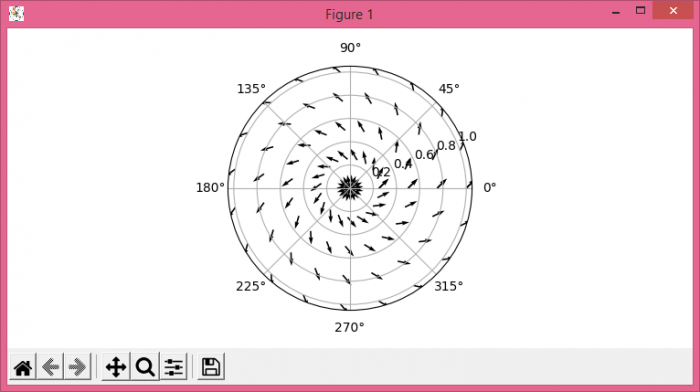# How to make a quiver plot in polar coordinates using Matplotlib?

To make a quiver plot in polar coordinates using Matplotlib, we can take the following steps −

• Set the figure size and adjust the padding between and around the subplots.

• Create radii, thetas, theta and r data points using numpy.

• Create a new figure or activate an existing figure.

• Add an 'ax' to the figure as part of a subplot arrangement.

• Make poly collections of arrows.

• To display the figure, use show() method.

## Example

import numpy as np
from matplotlib import pyplot as plt

plt.rcParams["figure.figsize"] = [7.50, 3.50]
plt.rcParams["figure.autolayout"] = True

thetas = np.linspace(0, 2 * np.pi, 20)

f = plt.figure()

plt.show()